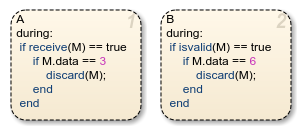Main Content

# isvalid

Determine if message is valid

## Syntax

``isvalid(message_name)``

## Description

example

````isvalid(message_name)` checks if an input or local message is valid. A message is valid if the chart has removed it from the queue and has not forwarded or discarded it.```

## Examples

expand all

When state `A` is active, receive message `M`. If the message has a data value equal to 3, discard the message. Then, when state `B` is active, check that the message `M` is still valid. If the message is valid and has a data value equal to 6, discard the message.

In state `A`:

```during: if receive(M) == true if M.data == 3 discard(M); end end```

In state `B`:

```during: if isvalid(M) == true if M.data == 6 discard(M); end end```## See Also

Introduced in R2018b

## SupportGet trial now